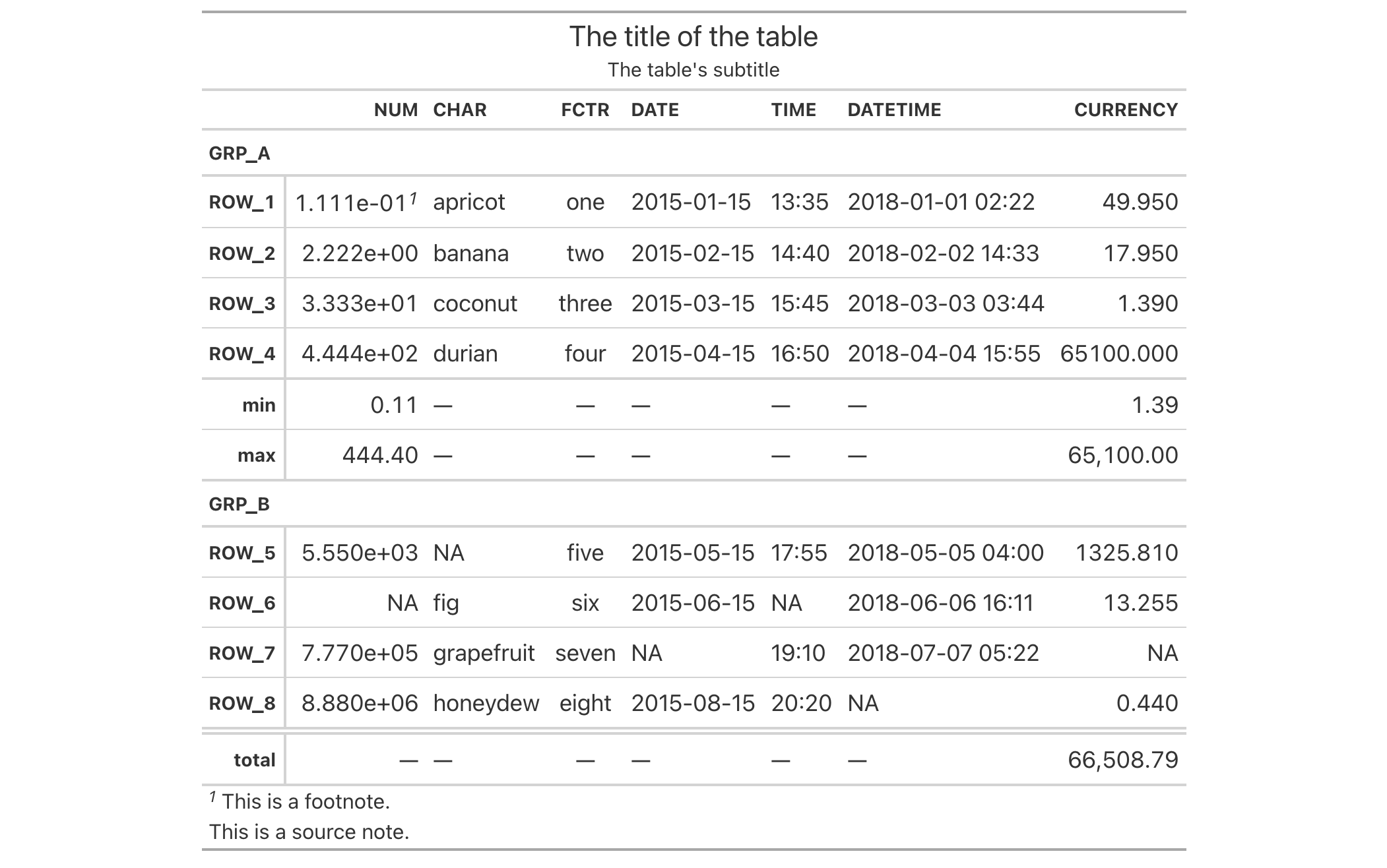Sometimes an all-capitalized look is suitable for a table. With the opt_all_caps() function, we can transform characters in the column labels, the stub, and in all row groups in this way (and there's control over which of these locations are transformed).

This function serves as a convenient shortcut for <gt_tbl> %>% tab_options(<location>.text_transform = "uppercase", <location>.font.size = pct(80), <location>.font.weight = "bolder") (for all locations selected).

## Usage

opt_all_caps(
data,
all_caps = TRUE,
locations = c("column_labels", "stub", "row_group")
)

## Arguments

data

A table object that is created using the gt() function.

all_caps

A logical value to indicate whether the text transformation to all caps should be performed (TRUE, the default) or reset to default values (FALSE) for the locations targeted.

locations

Which locations should undergo this text transformation? By default it includes all of the "column_labels", the "stub", and the "row_group" locations. However, we could just choose one or two of those.

## Value

An object of class gt_tbl.

## Examples

Use exibble to create a gt table with a number of table parts added. All text in the column labels, the stub, and in all row groups is to be transformed to all caps using opt_all_caps().

exibble %>%
gt(rowname_col = "row", groupname_col = "group") %>%
summary_rows(
groups = "grp_a",
columns = c(num, currency),
fns = list(
min = ~min(., na.rm = TRUE),
max = ~max(., na.rm = TRUE)
)) %>%
grand_summary_rows(
columns = currency,
fns = list(
total = ~sum(., na.rm = TRUE)
)) %>%
tab_source_note(source_note = "This is a source note.") %>%
tab_footnote(
footnote = "This is a footnote.",
locations = cells_body(columns = 1, rows = 1)
) %>%
title = "The title of the table",
subtitle = "The table's subtitle"
) %>%
opt_all_caps()## Function ID

9-6

Other table option functions: opt_align_table_header(), opt_css(), opt_footnote_marks(), opt_horizontal_padding(), opt_row_striping(), opt_stylize(), opt_table_font(), opt_table_lines(), opt_table_outline(), opt_vertical_padding()